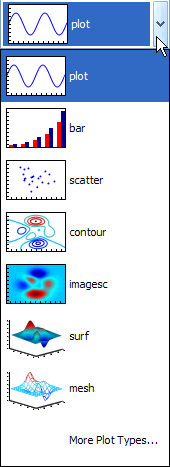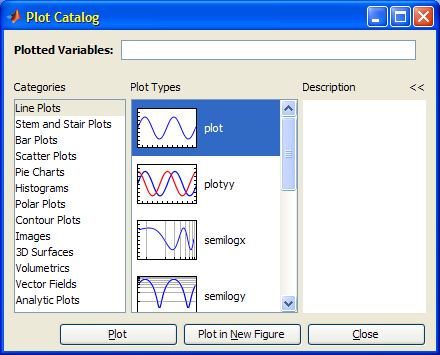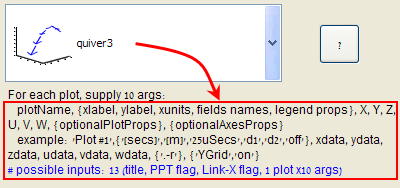# Plot-type selection components

Last week, Scott Hirsh, Matlab’s lead product manager, posted an article about Matlab’s redesigned plot catalog. In that post, Scott mentioned the internal deliberations about whether the plot catalog was needed at all. He explained how the final decision was to keep and enhance the catalog, rather than remove it, based on user feedback that the catalog is in fact useful, its main benefit being the fact that it is large and easy to use.

I posted a short comment mentioning my experience of using the catalog programmatically as another use-case that might be taken into consideration. Today, I would like to expand that short comment and explain how built-in plot-selection components can be used in our Matlab GUI programs.

### Built-in plot selection components

`PlotTypeCombo` is a plot-function selector is that is one of my personal favorite controls for embedding in a Matlab application:

```% Present the PlotCombo control import com.mathworks.mlwidgets.graphics.* jPlotCombo = PlotTypeCombo; pos = [100,100,170,50]; [jhPlotCombo,hPanel] = javacomponent(jPlotCombo,pos,gcf); set(jhPlotCombo,'ActionPerformedCallback',@myCBFcn);   % Callback function to process PlotCombo selections function myCBFcn(jObject,jEventData) newPlotFunc = jObject.getSelectedItem.getName.char; %Now do something useful with the selected function end % myCBFcn```

`PlotCatalog` is a dialog window that presents the plot functions catalog:

`com.mathworks.mlwidgets.graphics.PlotCatalog.getInstance.show`PlotTypeCombo and PlotCatalog

### Usage example

For one application that I developed for a client, I implemented a dynamic report page that enables users to select the plotting function using a `PlotTypeCombo`. When users select a non-default function (e.g. stairs or loglog), that function is automatically added to the combo-box for possible future reuse. I implemented this as follows:

```% Add the specified plotFunc to a PlotTypeCombo control function updatePlotCombo(plotCombo, plotFunc)   % Convert plotFunc (a Matlab string) into a Java PlotSignature import com.mathworks.mlwidgets.graphics.* plotSig = PlotMetadata.getPlotSignature(plotFunc);   % Get the list of all existing plot types in the combo box existingPlotTypes = {}; for plotIdx = 0 : plotCombo.ItemCount-1 nextItem = plotCombo.getItemAt(plotIdx); if isjava(nextItem) nextItem = char(nextItem.getName); end existingPlotTypes = [existingPlotTypes, nextItem]; end   % If the new plotType is NOT already in the list if isempty(strmatch(plotType,existingPlotTypes,'exact')) % Add the new plotType to the list just prior to the end, % so that "More plots..." will always be last plotCombo.insertItemAt(plotSig,plotCombo.ItemCount-1); end   % Set the currently-selected item to be the requested plotType % Note: temporarily disable callbacks to prevent involuntary action plotCombo.ActionPerformedCallback = []; plotCombo.setSelectedItem(plotSig); plotCombo.ActionPerformedCallback = @myCBFcn; % selection callback end % updatePlotCombo```

Usage of this function would then be as simple as invoking the updatePlotCombo() function:

`updatePlotCombo(jhPlotCombo,'stairs');`Dynamic plot selection example

### Plot signatures

The astute reader will have noticed from the updatePlotCombo() function above, that the `PlotTypeCombo` items are plot-signature objects. Different plot functions expect a different set of input arguments (a.k.a. signature). This information is kept and can be queried from the `PlotMetadata` class:

```>> com.mathworks.mlwidgets.graphics.PlotMetadata.listAllSignatures ans = [area, bar, bar (stacked), barh (stacked), barh, comet, compass, errorbar, feather, loglog, plot, plot N series, plot N series against T, plot3, plotmatrix, plotyy, polar, quiver, quiver3, ribbon, scatter, scatter3, semilogx, semilogy, stairs, stem, stem3, null, contour, contour3, contourf, image, imagesc, mesh, meshc, meshz, pcolor, plot against first column, surf, surfc, surfl, waterfall, null, contour, contour3, contourf, image, imagesc, mesh, meshc, meshz, pcolor, plot against first column, surf, surfc, surfl, waterfall]   >> plotFunc = 'surf'; % for example >> plotSig = PlotMetadata.getPlotSignature(plotFunc); >> args = cell(plotSig.getArgs)'; args = [1x1 com.mathworks.mlwidgets.graphics.PlotArgDescriptor] [1x1 com.mathworks.mlwidgets.graphics.PlotArgDescriptor] [1x1 com.mathworks.mlwidgets.graphics.PlotArgDescriptor] [1x1 com.mathworks.mlwidgets.graphics.PlotArgDescriptor]   >> argsStruct = []; % initialize >> for argsIdx = 1 : length(args) argsStruct(argsIdx).axis = char(args{argsIdx}.getAxis); argsStruct(argsIdx).name = char(args{argsIdx}.getName); argsStruct(argsIdx).label = char(args{argsIdx}.getLabel); argsStruct(argsIdx).dims = args{argsIdx}.getNumDimensions; argsStruct(argsIdx).reqObj = args{argsIdx}.getRequired; req = argsStruct(argsIdx).reqObj; argsStruct(argsIdx).requiredFlag = req.equals(req.REQUIRED); end   >> disp(argsStruct(1)) % Info about the first input argument axis: 'X' name: 'X' label: 'X Data Source' dims: [2x1 int32] reqObj: [1x1 com.mathworks.mlwidgets.graphics.PlotArgDescriptor\$RequiredType] requiredFlag: 0   >> disp({argsStruct.axis}) % axis info of all input arguments 'X' 'Y' 'Z' ''   >> disp({argsStruct.name}) % name of all input arguments 'X' 'Y' 'Z' 'C'   >> disp({argsStruct.label}) % data label of all input arguments 'X Data Source' 'Y Data Source' 'Z Data Source' 'Color'   >> disp({argsStruct.dims}) % dimensionality of all input args [2x1 int32] [2x1 int32]     >> disp({argsStruct.requiredFlag}) % is any input arg mandatory?    ```

This signature data can be queried for each separate plot type. In fact, I use this mechanism above so that whenever the user changes the plot-type in the `PlotTypeCombo`, the usage label text beneath the combo-box is updated with the specific plot’s signature information:Dynamic plot signature dataPrint

### 5 Responses to Plot-type selection components

1.Amjad Elshenawy says:

I am following the posts utilizing the package “com.mathworks.mlwidgets.graphics” with great interest.
The posts “Plot-type selection components” & “Color selection components” moved my thoughts towards the question: how can I list all classes of the package “com.mathworks.mlwidgets.graphics”?

Thanks Yair

•Yair Altman says:

@Amjad – simply open the file %matlabroot%\java\jar\mlwidgets.jar with your favorite zip application (WinZip or WinRAR, for example). A JAR file is just a simple ZIP file that contains the separate Java class files.

2.Brad Stiritz says:

Yair,

Are there any good options for restricting the set of plot types presented in the dialog? In many GUI contexts where the underlying data structure / types are fixed & well-known, I can imagine it wouldn’t make sense to present the entire catalog. Also, do you know if it’s possible to add custom plot types to the presented catalog?

Thanks,
•Yair Altman says:
@Brad – as I told you at the conference last week, you can indeed customize which plot types you wish to appear in the `PlotTypeCombo` – take a look at page 285 of my book.